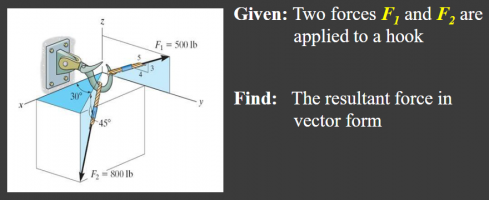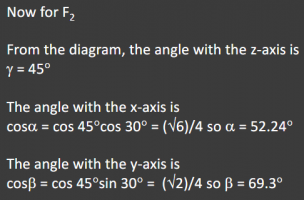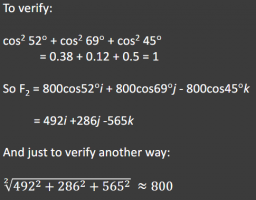# Find Direction Cosine from Planar Angles

#### isquaredplusone

##### New member
Hello,

apologies if my description is a bit hard to follow - I'm not very familiar with this and I may not use the correct terminology.

I am trying to find the direction cosine alpha of a 3d vector, where I know the angles that vector describes on the x-y plane (30 degrees) and against the z-axis (i.e. direction angle gamma) (45 degrees).

I have a solution for cos(alpha) as follows:

Cos(alpha) = cos(45).cos(30) = 52.24 degrees

However, I don't understand why this equation gives the correct answer. I can see that the cos(45) is effectively bringing the vector up onto the x-y plane and the cos(30) is then bring it across to the x-axis, but why does their product then yield alpha?

Similarly, the direction cosine beta is given as:

Cos(beta) = cos(45).sin(30) = 69.3 degrees

Again, I can see how these two triangles relate the vector to the y-axis, but I don't understand why the product gives beta.Apologies if my description is unintelligible, but any help would be greatly appreciated,
ThanksLast edited:

#### Subhotosh Khan

##### Super Moderator
Staff member
Hello,

apologies if my description is a bit hard to follow - I'm not very familiar with this and I may not use the correct terminology.

I am trying to find the direction cosine alpha of a 3d vector, where I know the angles that vector describes on the x-y plane (30 degrees) and against the z-axis (i.e. direction angle gamma) (45 degrees).

I have a solution for cos(alpha) as follows:

Cos(alpha) = cos(45).cos(30) = 52.24 degrees

However, I don't understand why this equation gives the correct answer. I can see that the cos(45) is effectively bringing the vector up onto the x-y plane and the cos(30) is then bring it across to the x-axis, but why does their product then yield alpha?

Similarly, the direction cosine beta is given as:

Cos(beta) = cos(45).sin(30) = 69.3 degrees

Again, I can see how these two triangles relate the vector to the y-axis, but I don't understand why the product gives beta.

Apologies if my description is unintelligible, but any help would be greatly appreciated,
ThanksPlease post the original problem, along with any figures. A clean "image" will be preferable.

#### blamocur

##### Senior Member
A general question: are you trying to solve this problem, or are you trying to understand a supplied solution?

I am trying to find the direction cosine alpha of a 3d vector, where I know the angles that vector describes on the x-y plane (30 degrees) and against the z-axis (i.e. direction angle gamma) (45 degrees).

Which 3d vector? I can see only 2 vectors in the picture. Do you mean [imath]F_2[/imath]?

Cos(alpha) = cos(45).cos(30) = 52.24 degrees

Do you mean "alpha = 52.24 degrees" ? Because [imath]\cos\alpha \approx 0.6124[/imath]

#### blamocur

##### Senior Member
I really just want to know why the product of the two cosines or the cosine and the sine give the correct answer.
Multiplication of the length of [imath]F_2[/imath] by [imath]\cos 45^\circ[/imath] gives you the length of [imath]F_2[/imath]'s projection on the XY plane. Subsequent multiplications by [imath]\sin 30^\circ[/imath] and [imath]\cos 30^\circ[/imath] provide projections to Y and X axis respectively.

#### isquaredplusone

##### New member
Hi,

thanks for that, but I'm still not clear. There are no lengths in the equations given, just angles.

Thanks.

#### Subhotosh Khan

##### Super Moderator
Staff member
Hi,

the solutions I've given are for F2.

Yes, alpha = 52.24 degrees, my mistake.

Here's the solution as I've been given it:
View attachment 33203

I really just want to know why the product of the two cosines or the cosine and the sine give the correct answer.

Thanks.
Where is the diagram that the problem-solution refers to in the second line?#### blamocur

##### Senior Member
Hi,

thanks for that, but I'm still not clear. There are no lengths in the equations given, just angles.

Thanks.
"Lengths" is not really a good word here, I meant the absolute value magnitude of the vector which in the case of [imath]F_2[/imath] is 800 lb.

#### isquaredplusone

##### New member
"Lengths" is not really a good word here, I meant the absolute value magnitude of the vector which in the case of [imath]F_2[/imath] is 800 lb.
Hi,

thanks again, but I can see I'm not being clear enough with my question, for which I apologise.

All the magnitudes and how the cosine and sine functions relate them together I'm pretty much happy with (or at least, I think I am!).

What I don't understand, and what I was really hoping for an explanation of, is this;

"Why is it that, when you multiply cos(45) by cos(30), you get cos(alpha), where cos(alpha) is the direction cosine, i.e. the angle between the 3d vector F2 and the x-axis?"

My question doesn't even really have to relate to this particular problem, which is why I didn't post the diagram in the first place, i.e. "why would multiplying the cosine of two angles together result in the cosine of a third angle?"

I very much appreciate everybody joining in with this discussion taking the time to do so - thanks very much#### blamocur

##### Senior Member
What I don't understand, and what I was really hoping for an explanation of, is this;

"Why is it that, when you multiply cos(45) by cos(30), you get cos(alpha), where cos(alpha) is the direction cosine, i.e. the angle between the 3d vector F2 and the x-axis?"

I did my best to answer exactly this question in #5.

#### isquaredplusone

##### New member
Absolutely, and I very much appreciate you taking the time to do so - equally, I apologise for not being able to follow your explanation - my lack of comprehension reflects entirely on my poor understanding, not your attempt to explain the solution.

I can absolutely see how multiplication by cos(45), cos(30) and sin(30) would project the magnitude of the vector onto the respective axis - your explanation makes that very clear and I'm very grateful for that.

What I'm having trouble visualising (perhaps?) is how that projection then gives the angle, particularly as the solution I've been given has no magnitudes in it. Am I to presume that unit-vectors are being used here, so are not included in the equations?

I appreciate and sympathise with your frustration at my lack of comprehension, but I would be very grateful for your forbearance in offering, perhaps, just a little more explanation.

Thanks.

#### blamocur

##### Senior Member
No apologies necessary: our goal is to help you understand. The more questions you ask the more likely we are to figure out the missing links in your understanding and be more effective in our efforts.

Back to our cosines: cosines in your problem are ratios of projections' magnitudes to the magnitudes of the original vector.
The projection [imath]F_{XY}[/imath] of [imath]F_2[/imath] onto the XY plane has magnitude $|F_{XY}| = |F_2| \cos 45^\circ\approx 0.7071 |F_2|$.
The projection [imath]F_X[/imath] of [imath]F_{XY}[/imath] onto the X axis is the same as the projection of [imath]F_2[/imath] onto the X axis, and thus
$|F_X| = |F_{XY}|\cos 30^\circ = |F_2|\cos 45^\circ \cos 30^\circ$.
But we also know that [imath]|F_X| = |F_2| \cos\alpha[/imath] where [imath]\alpha[/imath] is the angle between [imath]F_2[/imath] and the X axis, which means that $\cos\alpha = \cos 45^\circ \cos 30^\circ$.

Note: I used [imath]F_X[/imath] and [imath]F_{XY}[/imath] for simplicity, but I should have used something like [imath]F_{2,X}[/imath] and [imath]F_{2,XY}[/imath] since there are two forces there.

But having said all that I want to emphasize that the value of [imath]\alpha[/imath] is not at all important for solving the problem. You only need to find the components [imath]F_{2,X}, F_{2,Y}[/imath] and [imath]F_{2,Z}[/imath], don't you?

•isquaredplusone

#### isquaredplusone

##### New member
Hello,

thanks very much for going through that blow by blow - how the magnitudes effectively cancelled out was the thing I just couldn't follow.

I think the solution given uses cosine vectors to prove a point;...where i, j and k are unit vectors, which I don't really see the benefit of, to be honest.

From this point, the component magnitudes of F2 can be added to those of F1 and the final resultant component force(s) on the hook can be determined.

Having only ever worked on 2d problems before, 3d is proving surprisingly heavy going!

I very much appreciate your time and patience on this - many thanks.#### blamocur

##### Senior Member
Hello,

thanks very much for going through that blow by blow - how the magnitudes effectively cancelled out was the thing I just couldn't follow.

I think the solution given uses cosine vectors to prove a point;

View attachment 33222

...where i, j and k are unit vectors, which I don't really see the benefit of, to be honest.

From this point, the component magnitudes of F2 can be added to those of F1 and the final resultant component force(s) on the hook can be determined.

Having only ever worked on 2d problems before, 3d is proving surprisingly heavy going!

I very much appreciate your time and patience on this - many thanks.Quite glad you worked it out!

...where i, j and k are unit vectors, which I don't really see the benefit of, to be honest.

If you remove the unit vectors your expression will be just a sum of numbers instead of vectors -- you would then end up with a meaningless number, wouldn't you?

Having only ever worked on 2d problems before, 3d is proving surprisingly heavy going!

You are not alone there